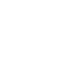304 North Cardinal St.
Dorchester Center, MA 02124

```function fibo( a ){
if ( a == 0 ){
return 0;
} else if ( a == 1 ) {
return 1;
}
return fibo( a ) + fibo( a-1 );
}```

```function fibo( a ){
if ( a == 1 || a == 2 ){
return 1;
}
if ( a == 0 ){
return 0;
}
return fibo( a-1 ) + fibo( a-2 );
}```

```function fibo(a){
if ( a == 0 ) return 0;
if ( a == 1 ) return 1;
return fibo( a - 1 )+fibo( a - 2 );
}```